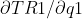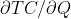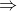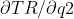In: Economics

# Consider a Cournot duopoly with the following inverse demand function:p(Q) =a−Q where p is the price...

Consider a Cournot duopoly with the following inverse demand function:p(Q) =a−Q where p is the price of the product and Q is the total amount of goods exchanged in the market. The total costs areC(q1) = 300q1, C(q2) = 300q2 for firm 1 and firm 2, respectively. But the demand is uncertain (i.e., a new product may be introduced soon which will decrease the demand drastically). Firm 1 learns whether demand will be high (a =1800) or small (a=900) before it makes its quantity decision. However, firm 2 knows just the probability of high demand (1/4) and the probability of low demand (3/4). All of this is common knowledge. In particular, firm 2 knows that firm 1 knows the demand for certain. The two firms simultaneously choose quantity. What is the Bayesian equilibrium of the game (price in both states of the world and quantity produced by each firm)?

## Solutions

##### Expert Solution

This question needs a bit to work out. It is been given that when firm 1 has a demand of 1800 the other has a demand of 900.So the demand function of say firm 1 can be written as:

P(Q)= 1800-Q

where Q= q1+q2

Total cost (TC)= 300q (for both under cournot duopoly model)

P= 1800-(q1+q2)

= 1800-q1-q2

Total revenue (TR1)= price *quantity(P(Q)*q1)

=(1800-q1-q2) q1

=1800q1-q12-q2q1

Now the marginal revenue(MR1)== 1800-2q1-q2

Marginal cost (MC)== 300

Since Cournot duopoly is operating at MR=MC, we have:

1800-2q1-q2=3002q1=1800-300-q22q1=1500-q2q1=750-1/2q2

Now firm 2 follows the same pattern:

P(Q)= 900-Q

where Q= q1+q2

Total cost (TC)= 300q (for both under cournot duopoly model)

P= 900-(q1+q2)

= 900-q1-q2

Total revenue (TR2)= price *quantity(P(Q)*q2)

=(900-q1-q2) q2

=900q1-q1q2-q2

Now the marginal revenue(MR1)== 900-q1-2q2

Marginal cost (MC)== 300

Since Cournot duopoly is operating at MR=MC, we have:

900-2q2-q1=3002q2=900-300-q12q2=600-q1q2=300-1/2q1

Substituting this to q1q1=750-1/2(300-1/2q1)q1=750-150+1/4q1600+1/4q1

q1=4/3*600

q1=800

substituting this to q2:

q2=300-1/2*800

q2=-100

q1 and q2 are the equilibrium quantities of firm 1 and firm 2 respectively at prices 1800 and 900.

## Related Solutions

##### 4. Consider a Cournot duopoly with inverse demand function P = 100 – Q. Firm 1’s...
4. Consider a Cournot duopoly with inverse demand function P = 100 – Q. Firm 1’s cost function is C1(q1) = 20q1, and firm 2’s cost function is C2(q2) = 30q2. Firms choose quantities once and simultaneously. (a) Write out each firm’s profit function. From these, derive the reaction functions of each firm, and solve for the Nash equilibrium quantities, price and profits. Illustrate your answer on a graph of the reaction functions (you do not need to draw isoprofit...
##### Consider a homogeneous good Cournot duopoly with inverse demand function given by p = 1 –...
Consider a homogeneous good Cournot duopoly with inverse demand function given by p = 1 – Q. The two firms have identical marginal costs equal to 0.4 and propose a merger. The firms claim that the merger will result in a decrease of the marginal cost of the merged firm by x per cent. How large would x need to be for welfare to increase rather than decrease as a result of the merger?
##### Let the equation of the inverse demand curve for a Cournot duopoly be p = 88...
Let the equation of the inverse demand curve for a Cournot duopoly be p = 88 - 2 (q1 + q2). where q1 is the output of firm 1 and q2 is the output of firm 2. Firm i's cost function is c(qi) = 8qi . (a)What are the Cournot duopoly equilibrium outputs, price and profit per firm for this market? (b) Suppose that for a market with the inverse demand equation and cost functions of part (a), firm 1...
##### The inverse market demand curve for a duopoly market is p=14-Q=14-q₁-q₂, where Q is the market...
The inverse market demand curve for a duopoly market is p=14-Q=14-q₁-q₂, where Q is the market output, and q₁ and q₂ are the outputs of Firms 1 and 2, respectively. Each firm has a constant marginal cost of 2 and a fixed cost of 4. Consequently, the Nash-Cournot best response curve for Firm 1 is q₁=6-q₂/2. A. Create a spreadsheet with Columns titled q₂, BR₁, Q, p, and Profit₁. In the first column, list possible quantities for Firm 2, q₂,...
##### a.) Two identical firms compete as a Cournot duopoly. The market demand is P=100-2Q, where Q...
a.) Two identical firms compete as a Cournot duopoly. The market demand is P=100-2Q, where Q stands for the combined output of the two firms, Q=q1 +q2. The marginal cost for each firm is 4. Derive the best-response functions for these firms expressing what q1 and q2 should be. b.) Continuing from the previous question, identify the price and quantity that will prevail in the Cournot duopoly market c.) Now suppose two identical firms compete as a Bertrand duopoly. The...
##### Two firms operate in a Cournot duopoly and face an inverse demand curve given by P...
Two firms operate in a Cournot duopoly and face an inverse demand curve given by P = 200 - 2Q, where Q=Q1+Q2 If each firm has a cost function given by C(Q) = 20Q, how much output will each firm produce at the Cournot equilibrium? a. Firm 1 produces 45, Firm 2 produces 45. b. Firm 1 produces 30, Firm 2 produces 30 c. Firm 1 produces 45, Firm 2 produces 22.5 d. None of the above.
##### The inverse market demand in a homogeneous-product Cournot duopoly is P = 140 – 2(Q1 +...
The inverse market demand in a homogeneous-product Cournot duopoly is P = 140 – 2(Q1 + Q2) and costs are Company 1, C1(Q1) = 18Q1 and Company 2 C2(Q2) = 17Q2. Calculate the equilibrium output for Company 1 Round all calculations to 1 decimal The inverse market demand in a homogeneous-product Cournot duopoly is P = 133 – 1(Q1 + Q2) and costs are Company 1, C1(Q1) = 10Q1 and Company 2 C2(Q2) = 21Q2. Calculate the equilibrium output for...
##### Consider the following inverse demand function, p(Q) = a-bQ, Q = q1 +q2, where a and...
Consider the following inverse demand function, p(Q) = a-bQ, Q = q1 +q2, where a and b are positive parameters and qi denotes firm i's output, i = 1, 2. Assume that the total cost of firm i is cqi2/2, with c > 0. Firms choose quantities simultaneously and non cooperatively (Cournot competition). The Cournot game described above is infinitely repeated. Firms use grim trigger strategies (infinite Nash reversion). Firms discount future profits at a rate r > 0. a)...
##### 3) (Symmetric Cournot) Consider a duopoly facing market demand p(Q) = 90 – 3Q, and assume...
3) (Symmetric Cournot) Consider a duopoly facing market demand p(Q) = 90 – 3Q, and assume each firm has cost function C(q) = 18q. For parts a-d, suppose these two firms engage in Cournot competition – that is, they simultaneously choose a quantity to produce, and then the price adjusts so that markets clear. [Recall that a firm’s Cournot best response function is the quantity that this firm will choose to produce in order to maximize its own profit, for...
##### Consider the Cournot competition example in the lecture notes. Inverse demand function is P(Q) = 31...
Consider the Cournot competition example in the lecture notes. Inverse demand function is P(Q) = 31 ? 2Q. However, make the change that firm B’s cost function is CB(Q) = 2Q. Firm A’s cost function remains the same at CA(Q) = Q. a) Determine firm A’s best response function Q*A (QB). b) Determine firm B’s best response function Q*B (QA). c) How much quantity is each firm producing in the Cournot d) What is the price at which output goods...